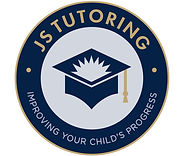# 11+ Maths Booster Webinars From JS Tutoring

## Six 11+ Maths Practice Papers with Answers and Recordings...

Would you like 6 11+ Maths Practice Papers (created by me from my 10 years' experience teaching 11+ Maths) with Answers and Recordings? Simply print out each Maths Practice Paper, then watch the recording, attempt them with your child and check against the answers provided.

Each Webinar begins with live teaching on KS2 Maths topics that always come up in the Maths section of the 11+ including other entrance tests. Watch me show you how to answer "30 Maths Questions in 60 Minutes".

• Webinar 1 - Practice Paper 1 (worksheets, recording and answers)

• Webinar 2 - Practice Paper 2 (worksheets, recording and answers)

• Webinar 3 - Practice Paper 3 (worksheets, recording and answers)

• Webinar 4 - Practice Paper 4 (worksheets, recording and answers)

• Webinar 5 - Practice Paper 5 (worksheets, recording and answers)

• Webinar 6 - Practice Paper 6 (worksheets, recording and answers) - and bringing together arithmetic and reasoning.

Only £7.50 per webinar.

Payment is required upfront for all 6 Webinars.

Each Webinar is approximately 1 hour.

Areas of Focus:

• Place Value & Rounding

• Addition & Subtraction

• Multiplication & Division

• Number Knowledge

• Multiplying & Dividing by 10, 100 and 1000

• Number Sequences

• Percentages, Fractions & Decimals

• Ratio & Proportion

• Algebra

• Mixed Calculations & Worded Problems

• Data Tables & Displaying Data

• The Mean, Median, Mode & Range

• Probability

• Angles

• 2D Shapes

• Area & Perimeter

• Symmetry

• 3D Shapes

• Coordinates

• Units

• Time